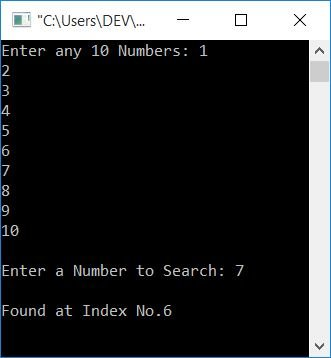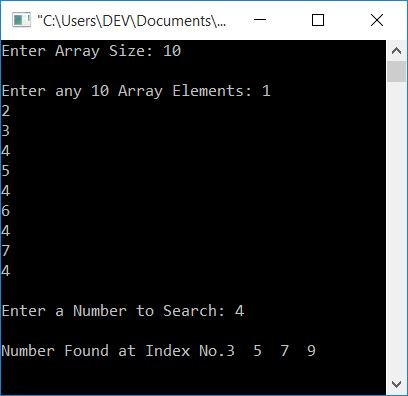# C Program for Linear Search

In this article, you will learn and get code for searching for a number or an element in a given array using the linear search technique. But before going through the program, if you want to check out the algorithm used for linear search, then refer to Linear Search.

## Linear Search in C

Linear search is the easiest way to search for an element in an array. It is also easy to learn. Let's first create a program for it. The question is: "Write a program in C that asks the user to enter any 10 array elements and then asks them to enter a number to search from the given array." The answer to this question is:

```#include<stdio.h>
#include<conio.h>
int main()
{
int arr, i, num, pos;
printf("Enter any 10 Numbers: ");
for(i=0; i<10; i++)
scanf("%d", &arr[i]);
printf("\nEnter a Number to Search: ");
scanf("%d", &num);
for(i=0; i<10; i++)
{
if(arr[i]==num)
{
pos=i;
break;
}
}
printf("\nFound at Index No.%d", pos);
getch();
return 0;
}```

This program was built and runs in the Code::Blocks IDE. Here is its sample run:Now supply any 10 numbers, say 1, 2, 3, 4, 5, 6, 7, 8, 9, and 10, and then enter a number, say 7, to search. When you press the ENTER key,Here is the output produced:As you can see from the above code, the number (stored in num) gets compared with each and every number one by one. That is, the first number (the number at the 0th index) gets compared to num. If found, then initialize the index to a variable, say pos, and with the help of the break keyword, exit the loop. Print the value of pos as the number's index. Otherwise, check for the number at the next index. Continue the process until the number is found.

Note: The previous program had two limitations. The first one is if the user enters a number that is not available in the list. The second is if the user enters a number that appears multiple times in the list or array. To overcome this problem, we have created another program.

## Linear Search with Duplicates in C

This program finds and prints the index number at which the number is available. If the given number is present in the given array in repeated order. The program then prints all of the indexes that are available.

```#include<stdio.h>
#include<conio.h>
int main()
{
int arr, size, i, num, arrTemp, j=0, count=0;
printf("Enter Array Size: ");
scanf("%d", &size);
printf("\nEnter any %d Array Elements: ", size);
for(i=0; i<size; i++)
scanf("%d", &arr[i]);
printf("\nEnter a Number to Search: ");
scanf("%d", &num);
for(i=0; i<size; i++)
{
if(arr[i]==num)
{
arrTemp[j] = i;
j++;
count++;
}
}
if(count>0)
{
printf("\nNumber Found at Index No.");
size = count;
for(i=0; i<size; i++)
printf("%d  ", arrTemp[i]);
}
else
getch();
return 0;
}```

Let's suppose the user has entered the array size as 10 and its 10 elements as 1, 2, 3, 4, 5, 4, 6, 4, 7, and 4. And the number to be found is 4. Therefore, here is its sample output, which you will see:Here the main logic used is:

• If the numbers coincide. Simply copy the current index number to another array, say arrTemp [].
• Each time, increment a variable, say count, by setting the index number in the array arrTemp []
• The array arrTemp[] holds index number(s) where the number is available, and the variable count holds the size of arrTemp[].

#### The same program in different languages

C Quiz

« Previous Program Next Program »